# ML Aggarwal Class 8 Solutions for ICSE Maths Chapter 4 Cubes and Cube Roots Check Your Progress

## ML Aggarwal Class 8 Solutions for ICSE Maths Chapter 4 Cubes and Cube Roots Check Your Progress

Question 1.
Show that each of the following numbers is a perfect cube. Also, find the number whose cube is the given number:
(i) 74088
(ii) 15625
Solution: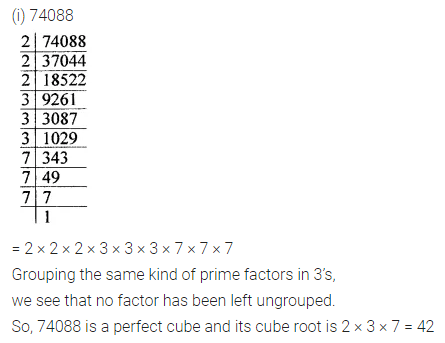Question 2.
Find the cube of the following numbers:
(i) -17
(ii) $$-3 \frac{4}{9}$$
Solution:Question 3.
Find the cube root of each of the following numbers by prime factorisation:
(i) 59319
(ii) 21952
Solution: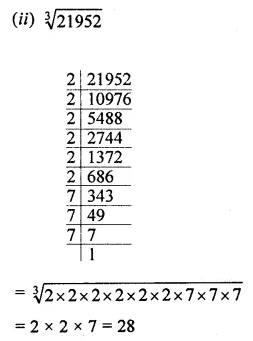Question 4.
Find the cube root of each of the following numbers:
(i) -9261
(ii) $$2 \frac{43}{343}$$
(iii) 0.216
Solution: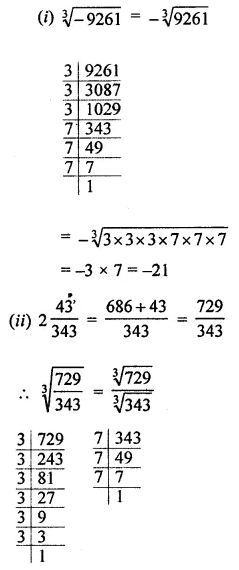Question 5.
Find the smallest number by which 5184 should be multiplied so that product is a perfect cube. Also find the cube root of the product.
Solution: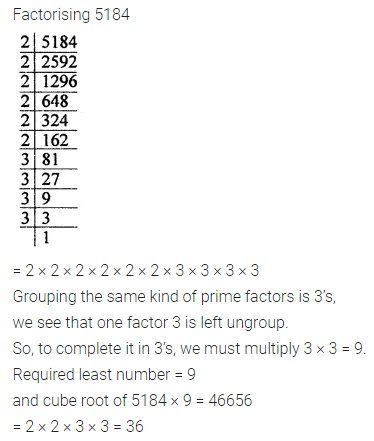Question 6.
Find the smallest number by which 8788 should be divided so that quotient is a perfect cube. Also, find the cube root of the quotient.
Solution: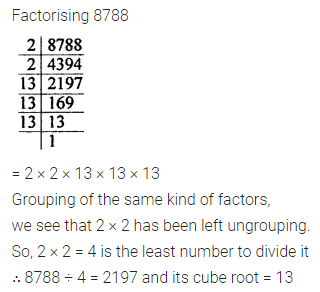Question 7.
Find the side of a cube whose volume is 4096 m3.
Solution: